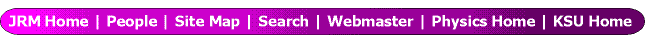DLD Anode Position Conversion This document is also available in MS Word or Adobe Acrobat formats. DLD Anode Position Conversion Kevin Carnes April 13, 2007     Derivation of Horizontal Position X   This development will be given in terms of the horizontal position signals.  Vertical position may be calculated in an analogous manner.  The horizontal signals coming from a DLD detector can be written, in units of ns, as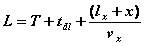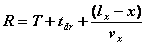Here,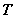is the time of arrival of the detected particle on the MCP,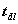and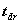are offsets, including the TOF from the MCP to the delayline anode (same for L and R) and any cable delay to the CFD (can be different for L and R) minus the cable and CFD delay for thesignal,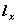is the half-length (in mm) of the horizontal winding on the delay line (i.e. the distance from the center to one end of the anode),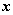is the position in mm from the center of the horizontal anode, and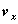is the velocity in mm/ns of the signal on the anode.  We can solve for the positionin mm as follows: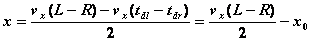,   where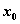is an offset to get the zero position correct.   With the LeCroy TDC, we actually measure the position signals in channels, where each channel corresponds to 0.5 ns.  Therefore, the full position calculation would be: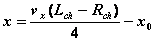,   where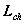and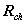are now in units of TDC channels.    Published Values of Transverse Velocity   The only way to definitively determine the constantsandis through the use of a detector mask with a calibrated grid.  However, Roentdek publishes approximate values (they say to within 5%) for each of their detectors that we can use as a check.  In the most recent version of their MCP Delay Line manual (v 6.2.90.7), they list what they call “single pitch propagation times” for each detector as follows:     DLD40            0.73 ns/mm DLD80            0.95 ns/mm DLD120          1.25 ns/mm   This number is the inverse of the propagation velocity.  Thus, the values forwould be:     DLD40= 1.37 mm/ns DLD80= 1.05 mm/ns DLD120= 0.80 mm/ns   Note that, assuming that the conversion from channels to ns has already been made, the position calculation can be made with an explicit factor of 2, as in, for the DLD80,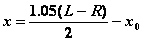,   or the conversion constant can be redefined to incorporate the factor of 2, as in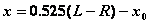The second form is equivalent to multiplying the Roentdek single pitch propagation time by 2, giving a value of 1.9 ns/mm for the DLD80, which corresponds to the number listed on page 8 of their manual as “the correspondence between position and time in the 2d image”.   Origin of Transverse Velocity and Consistency Checks   The values for the transverse velocities can be understood by looking at how a delayline is constructed.  We’ll use a DLD80 as an example.  The distance between the outer edges of the ceramic cylinders that hold the windings are 118 mm and 120 mm.  This works out to straight sections of 110 mm of wire in air on each side and 15 mm curved sections in contact with each cylinder.  If we consider a single loop of wire, this is 220 mm in air and 30 mm in contact with the cylinder.  The windings are in pairs, with the two wires of the pair .5 mm apart and a single wire wrapped 1 mm apart.  Therefore, for a single loop, the pitch is 250 mm to 1 mm.  A signal has to travel 250 mm around the loop to travel 1 mm in the transverse direction.  On the portion of the loop in air, the signal will travel at the speed of light.  However, according to Roentdek, the signal sees the ceramic on the turns and has an effective relative permittivity there of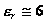, so that the propagation velocity is reduced by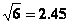.  Therefore, the time it takes the signal to go around one loop is: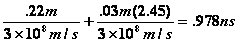.    This is .978 ns per 1 mm, or 1.02 mm/ns, close to the values of .95 ns and 1.05 mm/ns given in the Roentdek manual.  A consistency check on these values from actual data can made using time sum values.  We can sum the two position signals to get: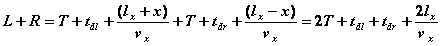The value we typically plot in our time sum spectra is therefore: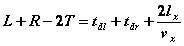If we take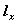to be one-half the length of a ceramic winding rod (one-half of 85 mm for the DLD80), and assuming thatandare neglible, the xdelay value should be roughly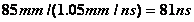.  Typically the two offsets will sum to a few nsec, increasing this value to give the measured time sum (the centroid of the peak in the time sum spectrum).   Conversion examples   Here are a few conversion examples from the sorting code I’m familiar with:   Itzik’s group:  Positions are calculated by a Posn function that takes as input a conversion factor Conv and the raw lsig and rsig signals in channels, then calculates   Posn = Conv*(1.0*lsig -1.0*rsig)/2.0   It uses a conversion value of Conv = ch2mmx = .51 mm/ch.  This is for a DLD80 detector.  So, one of the factors of 2 is included in the conversion constant and the other is explicit in the Posn calculation.   PAW FORTRAN code used by Lew and Igor’s groups:  Their resort code returns an array, typically called el for electrons and rec for recoils.  It is a two dimensional array.  The second dimension contains the hit number.  The first dimension has value 1 for the timing signal, 2 for the R – L signal, and 3 for the D – U signal.  In the analyse.f code, these values are converted to position in mm by multiplying by a conversion factor, gx or gy, and dividing by 2, as in xr1 = gxr*result(2,1)/2.  Note:  in some older code, this explicit factor of 2 was omitted, so that spectra plotting position in mm were incorrect.  Momenta values were corrected by including another factor of 2 in their calculation.  Instead of using a factor of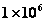to convert from mm/ns to m/s for the further conversion to au, a factor of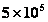was used.   Andre Staudte’s code:  He uses a function called makepos, where, for a DLD anode, the position is (tdc(x1) – tdc(x2))/(2.*f), where the tdc values are already in ns, and f is around 1. for a DLD80.   University of Colorado COBOLD code:  Inside a C++ function called AnalysisProcessEvent, they calculate position as DLD_pos_x = (tdc_ns(x1)-tdc_ns(x2))*scalefactor, where the scalefactor is .386.  Note the conversion from channels to ns has already been made, and the other factor of 2 is incorporated into the scalefactor (DLD120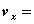0.80, so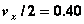).
 See the complete index of tutorials. Last updated on Tuesday, 21-Oct-2008.